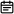# 152. 乘积最大子序列2019-06-06

## 题目链接

https://leetcode-cn.com/problems/maximum-product-subarray/

## 题目描述

``````输入: [2,3,-2,4]

``````输入: [-2,0,-1]

## 解题方案

### 思路

• 标签：动态规划
• 遍历数组时计算当前最大值，不断更新
• 令imax为当前最大值，则当前最大值为`imax = max(imax * nums[i], nums[i])`
• 由于存在负数，那么会导致最大的变最小的，最小的变最大的。因此还需要维护当前最小值imin，`imin = min(imin * nums[i], nums[i])`
• 当负数出现时则imax与imin进行交换再进行下一步计算
• 时间复杂度：O(n)

### 代码

``````class Solution {
public int maxProduct(int[] nums) {
int max = Integer.MIN_VALUE, imax = 1, imin = 1;
for(int i=0; i<nums.length; i++){
if(nums[i] < 0){
int tmp = imax;
imax = imin;
imin = tmp;
}
imax = Math.max(imax*nums[i], nums[i]);
imin = Math.min(imin*nums[i], nums[i]);

max = Math.max(max, imax);
}
return max;
}
}``````

### 画解